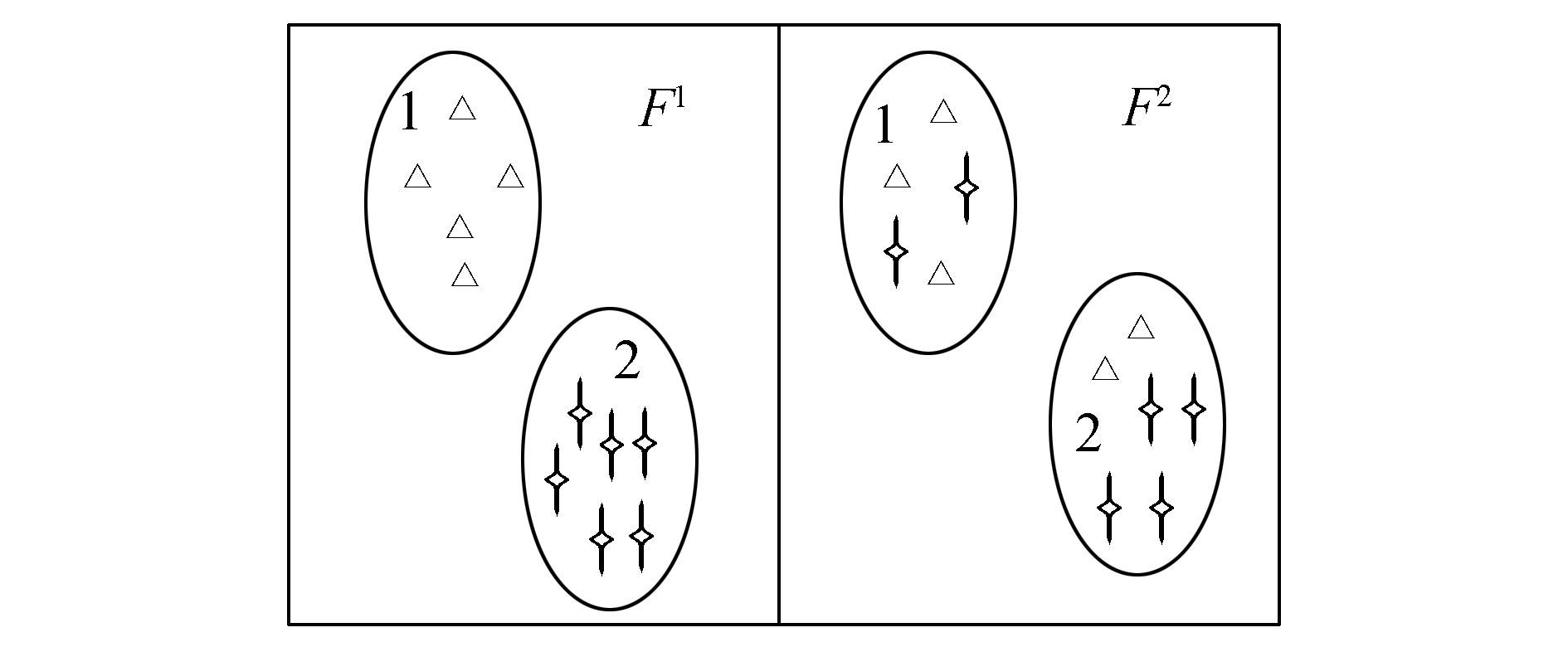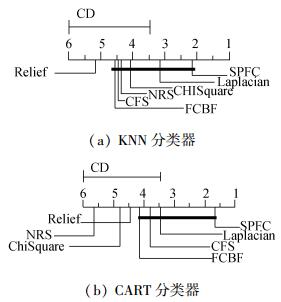﻿ 基于特征相关的谱特征选择算法
«上一篇文章快速检索 高级检索

 智能系统学报2017, Vol. 12Issue (4): 519-525  DOI: 10.11992/tis.2016090080

### 引用本文HU Minjie, LIN Yaojin, YANG Honghe, et al. Spectral feature selection based on feature correlation[J]. CAAI Transactions on Intelligent Systems, 2017, 12(4): 519-525. DOI: 10.11992/tis.201609008.### 文章历史

Spectral feature selection based on feature correlation
HU Minjie, LIN Yaojin, YANG Honghe, ZHENG Liping, FU Wei
School of Computer Science, Minnan Normal University, Zhangzhou 363000, China
Abstract: In the traditional spectrum feature selection algorithm, only the importance of single features are considered. In this paper, we introduce the statistical correlation between features into traditional spectrum analysis and construct a spectral feature selection model based on feature correlation. First, the proposed model utilizes the Laplacian Score to identify the most central feature as the selected feature, then designs a new feature group discernibility objective function, and applies the forward greedy search strategy to sequentially evaluate the candidate features. Then, the candidate feature with the minimum objective function is added to the selected features. The algorithm considers both the importance of feature as well as the correlations between features. We conducted experiments on two different classifiers and eight UCI datasets, the results of which show that the algorithm effectively improves the classification performance of the feature subset and also obtains a small number of feature subsets with high classification precision.
Key words: feature selection    spectral feature selection    spectral graph theory    feature relevance    discernibility    search strategy    Laplacian score    classification performance

1 谱特征选择算法

 $\mathit{\boldsymbol{S}} _{ij}= \left\{ \begin{array}{l} \frac 1 {{n}_{k}},\ \ {y}_{i}={y}_{j}=k\\ 0,\ \ 其他 \end{array} \right.$

G为一无向有权图，则邻接矩阵Wij=Sij(1≤i, jm), 且W为对称矩阵。令度矩阵D

 $\mathit{\boldsymbol{D}}= \left\{ \begin{array}{l} \sum\limits^{m} _{j=1} \mathit{\boldsymbol{W}}_{ji},\ \ j=i\\ 0,\ \ j\ne i \end{array} \right.$ (1)

 $\mathscr{L}=\mathit{\boldsymbol{D}}-\mathit{\boldsymbol{S}}, \mathscr{L}=\mathit{\boldsymbol{D}}^{-\frac 1 2} \mathit{\boldsymbol{LD}}^{-\frac 1 2}$

 $\text{let}\ \mathit{\boldsymbol{I}} ={[1 1 \cdots 1]}^\text{T}, \mathit{\boldsymbol{L}}*\mathit{\boldsymbol{I}}=0$

 $\forall \mathit{\boldsymbol{x}}∈\mathit{\boldsymbol{R}}^n, \mathit{\boldsymbol{x}}^\text{T}\mathit{\boldsymbol{Lx}} =\frac 1 2\sum\limits{ \mathit{\boldsymbol{w}}}_{ij}{ (\mathit{\boldsymbol{x}}_i- \mathit{\boldsymbol{x}} _j})^2$

 $\forall x∈{\mathit{\boldsymbol{R}}}^{n}, {\forall t∈R, (\mathit{\boldsymbol{x}}- \mathit{\boldsymbol{t}}*\mathit{\boldsymbol{e}}})^{\text{T}}L( \mathit{\boldsymbol{x}}-\mathit{\boldsymbol{t}}*\mathit{\boldsymbol{e}})={ \mathit{\boldsymbol{x}}}^{\text{T}} \mathit{\boldsymbol{Lx}}$图 1 特征与样本分布一致性示意图 Fig.1 The characteristics and the sample distribution consistency schematic diagram

 $φ(F^r)=\frac{\sum_{i,j}S_{ij}(f_{ri}-f_{rj})^2} {V(\mathit{\boldsymbol{f}}_r)}$ (2)

 $V(x)=∫\limits_ {M}{(x-u)}^2\text{d}P(x)$

 $V(\mathit{\boldsymbol{f}}_r)=\sum_i{(f_{ri}-u_r)}^2D_{ii}$

 $u_r=\sum_i \left( f_{ri}\frac{D_{ii}} {\sum_iD_{ii}} \right) =\frac{\sum_if_{ri}D_{ii}} {\sum_i{D}_{ii}}$

$∑_{i,j}S_{ij}(f_{ri}-f_{rj})^2$越小表示在样本分布结构图里近邻的样本在该特征上差异很小，即一个识别能力强的特征会使得Sij大而(fri-frj)小，因而式(2) 趋小。$∑_i(f_{ri}-u_r)^2D_{ii}$越大表示该特征在各样本上的取值方差越大，一个区分能力强的特征应该会赋予同类样本近似的值而不同类样本差异大的值，即具有较大方差的特征具有较强的识别能力。因此式（2）通过谱图理论结合特征的局部信息保持能力和方差来进行特征选择。

2 基于特征相关的谱特征选择模型

 $φ（\mathit{\boldsymbol{F}}_S）=\frac{\text{sqrt}(\sum\limits^k _{r=1}\sum_{i,j}(S_{ij}(f_{ri}-f_{rj})^2)} {\frac{\sum\limits^k _{r=1}\sum_i(f_{ri}-u_r)^2 D_{ii}} k}$ (3)

 $\text{argminRed}(\mathit{\boldsymbol{f}}_i)_{f_i∈F_U}=φ(\mathit{\boldsymbol{F}}_S∪\mathit{\boldsymbol{F}}_i)-φ(\mathit{\boldsymbol{F}}_S)$ (4)

1）FS=$\varnothing$FU=[F1 F2Fn]；

2）依据XY计算每两个样本间的相似度矩阵Sij(1≤i, jm);

3）依据相似度构建Laplacian图G，并计算WDL;

4）根据传统谱特征选择算法求出最具有识别力的一个特征fi

 $\mathit{\boldsymbol{F}}_S=\mathit{\boldsymbol{F}}_S∪\mathit{\boldsymbol{f}}_i，\mathit{\boldsymbol{F}}_U= \mathit{\boldsymbol{F}}_U-\{\mathit{\boldsymbol{f}}_i\};$

5）while FU不为空

6）根据式（3）计算φ(FS);

7）for i=1 to length(FU)

if (φ(FS)∪fi)-φ(FS))>0 then

L(j)=φ(FS)∪(fi)-φ(FS);

j=j+1;

end if

8) end for

9) 将L按升序排序

FS=FSfL(1)，FU=FU-{fL(1)};

10) end while

3 实验设计与对比 3.1 实验数据表 1 实验数据描述 Tab.1 Experimental data description
3.2 实验结果与分析表 2 不同特征选择算法在KNN分类器下的分类精度比较 Tab.2 Classification accuracies of feature selection with different algorithms based on KNN表 3 不同特征选择算法在CART分类器下的分类精度比较 Tab.3 Classification accuracies of feature selection with different algorithms based on CART

1）从总体上看，SPFC算法相比CFS、ChiSquare、FCBF、Laplacian、NRS以及Relief算法在KNN、CART基分类器下表现稳定，且均获得最高平均分类精度。相比考虑类信息的传统谱特征选择算法Laplacian, SPFC算法优于Laplacian。

2）相比ChiSquare、Laplacian、Relief这3种同样获得特征系列的算法，SPFC算法以相同的前k个特征在不同的基分类器下获得的平均分精度明显较高，相比子集约简的算法CFS、FCBF、NRS，SPFC取它们子集约简数量的最小值在两个基分类器下分类精度明显要高于NRS，在CART基分类器下SPFC的分类精度高于CFS、FCBF达两个百分点以上，而在KNN基分类器下也显著高于CFS、FCBF。

3）每一种算法均会在某一个分类器上某个数据集上获得最高分类精度，但只有SPFC能在两个基分类器上多个数据集上获得最高分类精度。SPFC算法在数据集ICU、rice、zoo上性能提升更为明显，在两个分类器上均达到最高。而ICU为混合型数据，rice为连续型数据、zoo为离散型数据。说明SPFC可以处理多类型数据集，在大部分各类型数据集上SPFC均能达到较好的稳定表现。

 $x^2_F=\frac{12N} {k(k+1)} \left[ \sum\limits^{k} _{i=1} {R}^2_i-\frac{k{(k+1)}^2} 4\right]\\ F_F=\frac{(N-1)x^2_F} {N(k-1)-x^2_F}$ (5)

 ${\text{CD}}_α=q_α \sqrt{\frac{k(k+1)} {6N}}$表 4 不同算法在两个分类器下的排序均值表 Tab.4 Different algorithms under the two classifiers the mean of sorting table图 2 在KNN和CART分类器下SPEC与其他算法的比较 Fig.2 SPEC compared with other algorithms Under the CART and KNN classifier
4 结论

  LIN Yaojin, Li Jinjin, LIN Peirong, et al. Feature selection via neighborhood multi-granulation fusion[J]. Knowledge-based systems, 2014, 67: 162-168. DOI:10.1016/j.knosys.2014.05.019 (0)  MANORANJAN D, LIU Huan. Consistency-based search in feature selection[J]. Artificial intelligence, 2003, 151(1): 155-176. (0)  ZHANG C, ARUN K, CHRISTOPHER R. Materialization optimizations for feature selection workloads[J]. ACM transactions on database systems, 2016, 41(1): 2. (0)  曹晋, 张莉, 李凡长. 一种基于支持向量数据描述的特征选择算法[J]. 智能系统学报, 2015, 10(2): 215-220. CAO Jin, ZHANG li, LI Fanchang. A feature selection algorithm based on support vector data description[J]. CAAI transactions on intelligent systems, 2015, 10(2): 215-220. (0)  MANORANJAN D, LIU Huan. Feature selection for classification[J]. Intelligent data analysis, 1997, 1(3): 131-156. (0)  SUN Yujing, WANG Fei, WANG Bo, et al. Correlation feature selection and mutual information theory based quantitative research on meteorological impact factors of module temperature for solar photovoltaic systems[J]. Energies, 2016, 10(1): 7. DOI:10.3390/en10010007 (0)  CVETKOVIC D M, ROWLINSON P. Spectral graph theory[J]. Topics in algebraic graph theory, 2004: 88-112. (0)  ZHAO Zheng, LIU Huan. Spectral feature selection for supervised and unsupervised learning[C]//Proceedings of the 24th international conference on Machine learning. ACM, 2007:1151-1157. (0)  ZHAO Zhou, HE Xiaofei, CAI Deng, et al. Graph regularized feature selection with data reconstruction[J]. IEEE transactions on knowledge and data engineering, 2016, 28(3): 689-700. DOI:10.1109/TKDE.2015.2493537 (0)  HE Xiaofei, CAI Deng, NIYONGI P. Laplacian score for feature selection[M]. Cambridge: MIT Press, MA, 2005: 507-514. (0)  BELABBAS M A, WOLFE P J. Spectral method in machine learning and new strategies for very large datasets[J]. Proceedings of the national academy of sciences, 2009, 106(2): 369-374. DOI:10.1073/pnas.0810600105 (0)  WANG Xiaodong, ZHANG Xu, ZENG Zhiqiang, et al. Unsupervised spectral feature selection with l 1-norm graph[J]. Neurocomputing, 2016, 200: 47-54. DOI:10.1016/j.neucom.2016.03.017 (0)  边肇祺, 张学工. 模式识别[M]. 2版. 北京: 清华大学出版社, 2000. (0)  HALL M A. Correlation-based feature selection for discrete and numeric class machine learning[C]//the 17th International Conference on Machine Learning. San Francisco:Morgan Kaufmann, 2000:359-366. (0)  ANDREAS W, ANDREAS P. Attacks on stegan ographic systems[M]. Heidelberg, Berlin: Springer-Verlag, 2000: 61-76. (0)  YU Lei, LIU Huan. Efficient feature selection via analysis of relevance and redundancy[J]. Journal of machine learning research, 2004, 5(1): 1205-1224. (0)  HU Qinghua, YU Daren, LIU Jinfu, et al. Neighborhood rough set based heterogeneous feature subset selection[J]. Information sciences, 2008, 178(18): 3577-3594. DOI:10.1016/j.ins.2008.05.024 (0)  CRAMMER K, GILAD-BACHRACH R, NAVOT A. Margin analysis of the lvq algorithm[C]//Advances in Neural Information Processing Systems. 2002, 14:462-469. (0)  FRIEDMAN M. A comparison of alternative tests of significance for the problem of m rankings[J]. The annals of mathematical statistics, 1940, 11(1): 86-92. DOI:10.1214/aoms/1177731944 (0)  DUNN O J. Multiple comparisons among means[J]. Journal of the american statistical association, 1961, 56(293): 52-64. DOI:10.1080/01621459.1961.10482090 (0)